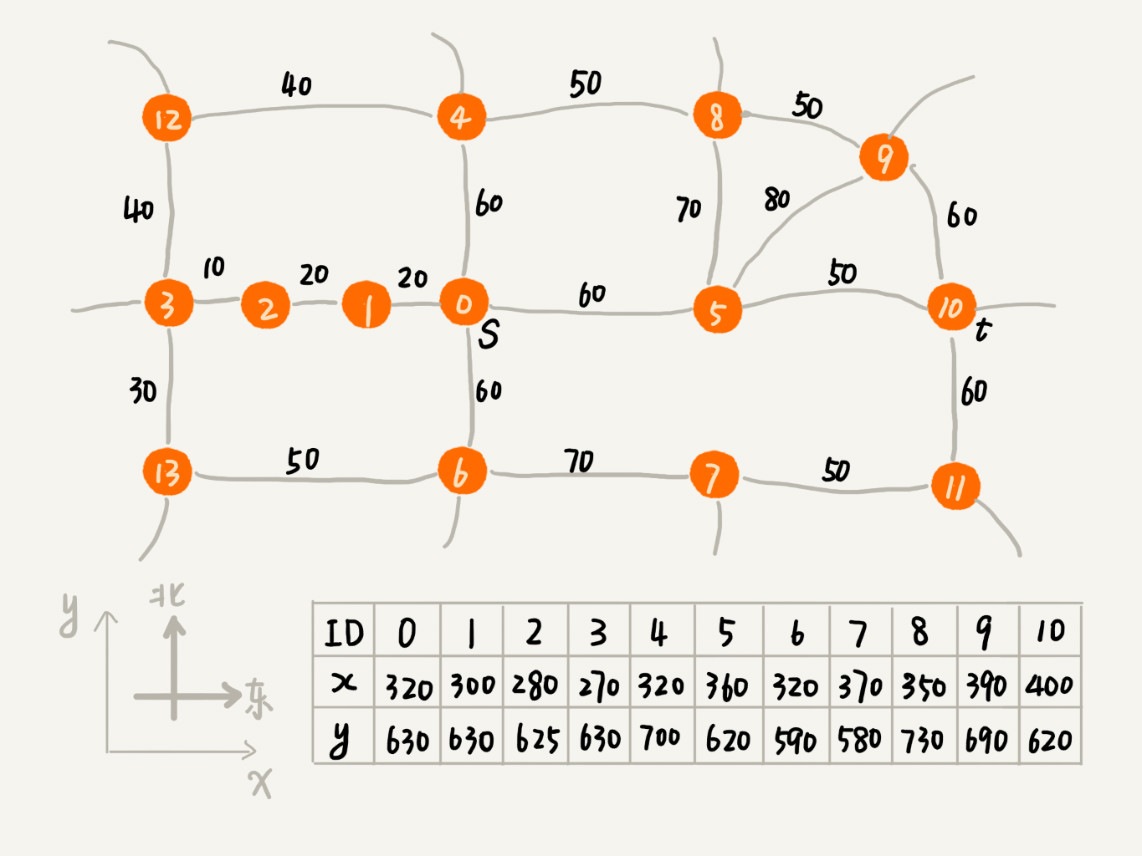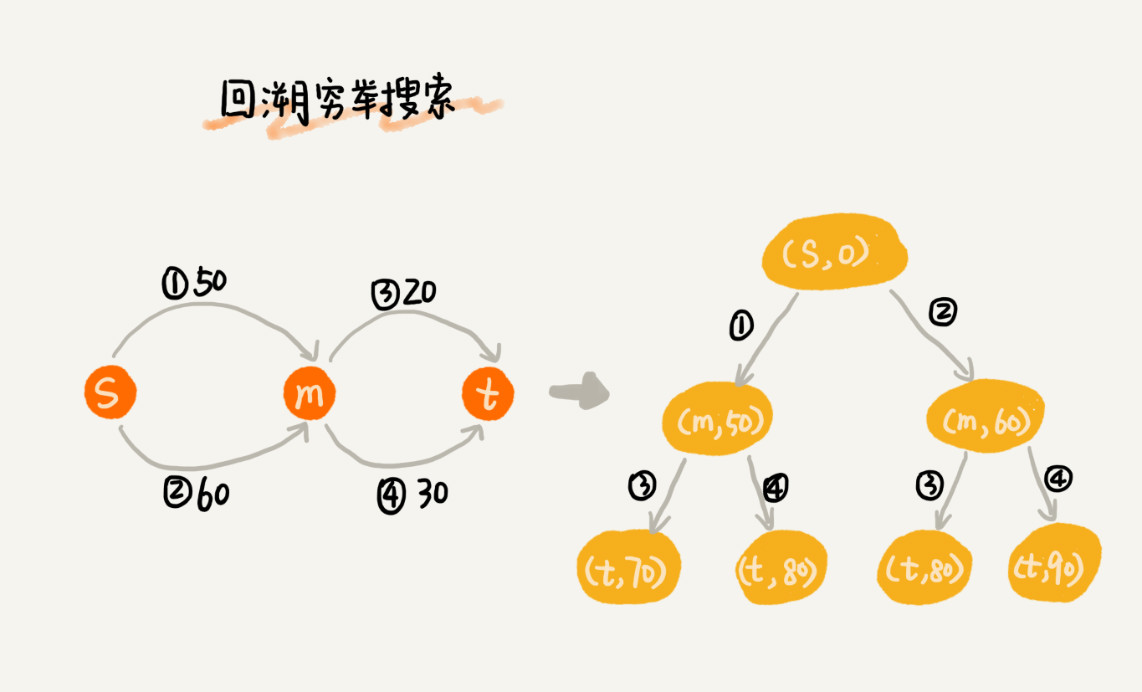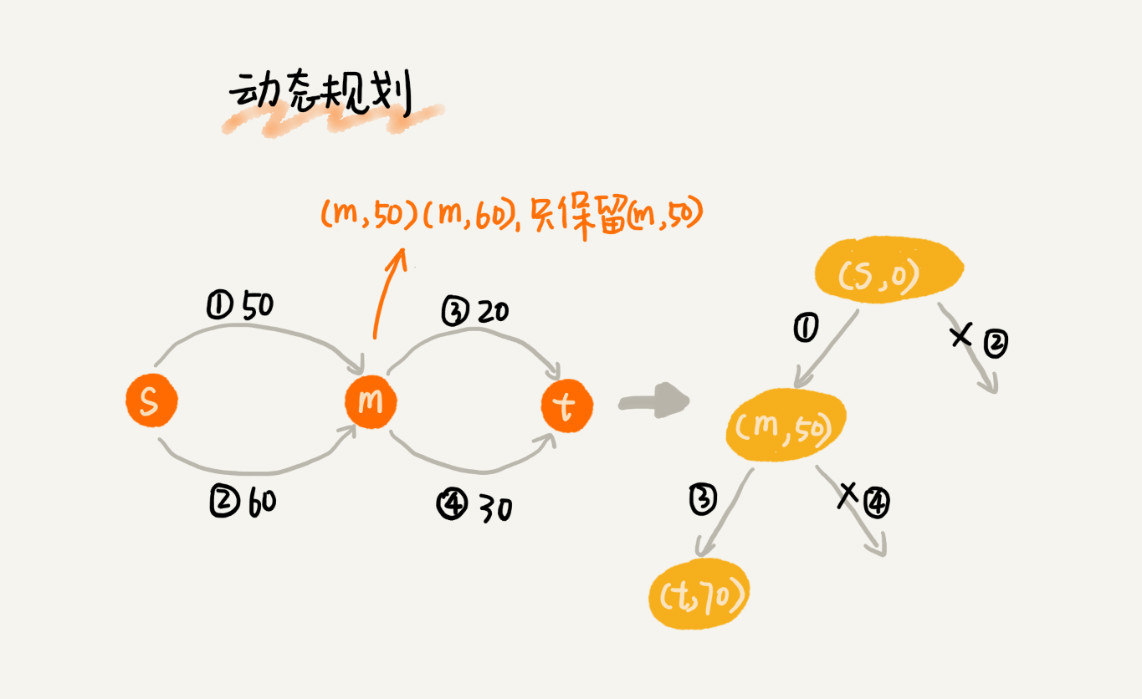## 算法解析

`Dijkstra` 算法有点儿类似 `BFS` 算法，它每次找到跟起点最近的顶点，往外扩展。这种往外扩展的思路，其实有些盲目。为什么这么说呢？举一个例子来解释一下。下面这个图对应一个真实的地图，每个顶点在地图中的位置，用一个二维坐标`(x,y)`来表示，其中，`x` 表示横坐标，`y` 表示纵坐标。`Dijkstra` 算法的实现思路中，用一个优先级队列，来记录已经遍历到的顶点以及这个顶点与起点的路径长度。顶点与起点路径长度越小，就越先被从优先级队列中取出来扩展，从图中举的例子可以看出，尽管找的是从 `s``t` 的路线，但是最先被搜索到的顶点依次是 `1``2``3`。通过肉眼来观察，这个搜索方向跟期望的路线方向（`s``t` 是从西向东）是反着的，路线搜索的方向明显“跑偏”了。

``````int hManhattan(Vertex v1, Vertex v2) { // Vertex 表示顶点，后面有定义
return Math.abs(v1.x - v2.x) + Math.abs(v1.y - v2.y);
}``````

`A*` 算法的代码实现中，顶点 `Vertex` 类的定义，跟 `Dijkstra` 算法中的定义，稍微有点儿区别，多了 `x``y` 坐标，以及刚刚提到的 `f(i)` 值。图 `Graph` 类的定义跟 `Dijkstra` 算法中的定义一样。

``````private class Vertex {
public int id; // 顶点编号 ID
public int dist; // 从起始顶点，到这个顶点的距离，也就是 g(i)
public int f; // 新增：f(i)=g(i)+h(i)
public int x, y; // 新增：顶点在地图中的坐标（x, y）
public Vertex(int id, int x, int y) {
this.id = id;
this.x = x;
this.y = y;
this.f = Integer.MAX_VALUE;
this.dist = Integer.MAX_VALUE;
}
}
// Graph 类的成员变量，在构造函数中初始化
Vertex[] vertexes = new Vertex[this.v];
// 新增一个方法，添加顶点的坐标
public void addVetex(int id, int x, int y) {
vertexes[id] = new Vertex(id, x, y)
}``````

`A*` 算法的代码实现的主要逻辑是下面这段代码。它跟 `Dijkstra` 算法的代码实现，主要有 `3` 点区别：

• 优先级队列构建的方式不同。`A*` 算法是根据 `f` 值（也就是刚刚讲到的 `f(i)=g(i)+h(i)`）来构建优先级队列，而 `Dijkstra` 算法是根据 `dist` 值（也就是刚刚讲到的 `g(i)`）来构建优先级队列；

• `A*` 算法在更新顶点 `dist` 值的时候，会同步更新 `f` 值；

• 循环结束的条件也不一样。`Dijkstra` 算法是在终点出队列的时候才结束，`A*` 算法是一旦遍历到终点就结束。

``````public void astar(int s, int t) { // 从顶点 s 到顶点 t 的路径
int[] predecessor = new int[this.v]; // 用来还原路径
// 按照 vertex 的 f 值构建的小顶堆，而不是按照 dist
PriorityQueue queue = new PriorityQueue(this.v);
boolean[] inqueue = new boolean[this.v]; // 标记是否进入过队列
vertexes[s].dist = 0;
vertexes[s].f = 0;
inqueue[s] = true;
while (!queue.isEmpty()) {
Vertex minVertex = queue.poll(); // 取堆顶元素并删除
for (int i = 0; i < adj[minVertex.id].size(); ++i) {
Edge e = adj[minVertex.id].get(i); // 取出一条 minVetex 相连的边
Vertex nextVertex = vertexes[e.tid]; // minVertex-->nextVertex
if (minVertex.dist + e.w < nextVertex.dist) { // 更新 next 的 dist,f
nextVertex.dist = minVertex.dist + e.w;
nextVertex.f
= nextVertex.dist+hManhattan(nextVertex, vertexes[t]);
predecessor[nextVertex.id] = minVertex.id;
if (inqueue[nextVertex.id] == true) {
queue.update(nextVertex);
} else {
inqueue[nextVertex.id] = true;
}
}
if (nextVertex.id == t) { // 只要到达 t 就可以结束 while 了
queue.clear(); // 清空 queue，才能退出 while 循环
break;
}
}
}
// 输出路径
System.out.print(s);
print(s, t, predecessor); // print 函数请参看 Dijkstra 算法的实现
}```````Dijkstra` 算法在此基础之上，利用动态规划的思想，对回溯搜索进行了剪枝，只保留起点到某个顶点的最短路径，继续往外扩展搜索。动态规划相较于回溯搜索，只是换了一个实现思路，但它实际上也考察到了所有从起点到终点的路线，所以才能得到最优解。`A*` 算法之所以不能像 `Dijkstra` 算法那样，找到最短路径，主要原因是两者的 `while` 循环结束条件不一样。刚刚提到，`Dijkstra` 算法是在终点出队列的时候才结束，`A*` 算法是一旦遍历到终点就结束。对于 `Dijkstra` 算法来说，当终点出队列的时候，终点的 `dist` 值是优先级队列中所有顶点的最小值，即便再运行下去，终点的 `dist` 值也不会再被更新了。对于 `A*` 算法来说，一旦遍历到终点，就结束 `while` 循环，这个时候，终点的 `dist` 值未必是最小值。

`A*` 算法利用贪心算法的思路，每次都找 `f` 值最小的顶点出队列，一旦搜索到终点就不在继续考察其他顶点和路线了。所以，它并没有考察所有的路线，也就不可能找出最短路径了。

## 总结引申

`A*` 算法属于一种启发式搜索算法`Heuristically Search Algorithm`）。实际上，启发式搜索算法并不仅仅只有 `A*` 算法，还有很多其他算法，比如 `IDA*` 算法、蚁群算法、遗传算法、模拟退火算法等。